# Splay(伸展树)

## 0. 准备工作

1. 神码是 Dfs 序
2. 神码是二叉树的旋转
3. 二叉树旋转之后为什么中序遍历不变 (极其重要)
4. 线段树区间操作是是什么原理

## 1.Splay 是神码

Splay 是一种最优秀比较优秀的数据结构，它支持很多操作 (几乎你想得到它就做得到)，但是缺点就是常数较大。
Splay 满足二叉搜索树的很多性质：

1. 有序性：伸展树中的每一个节点的值都大于左儿子，小于右儿子
2. 中序遍历不变性：伸展树无论经过怎样的旋转，中序遍历总是不变的，而且中序遍历肯定是升序遍历 (或者你也可以让他降序)
3. 自我调整性：简单说就是旋旋更健康：操作之后 Splay() 一下就可以维护树的健康度在 logn 左右 (每次操作的复杂度)，至于 Splay 是什么操作，待会会讲

## 2. 如何实现一棵简单的 Splay

#### 首先，我们需要明确几个操作，以及它们的作用 。#### 现在，我们需要将 x 节点向上转移，我们可以利用 zig 和 zag 操作。但是这也分多种情况。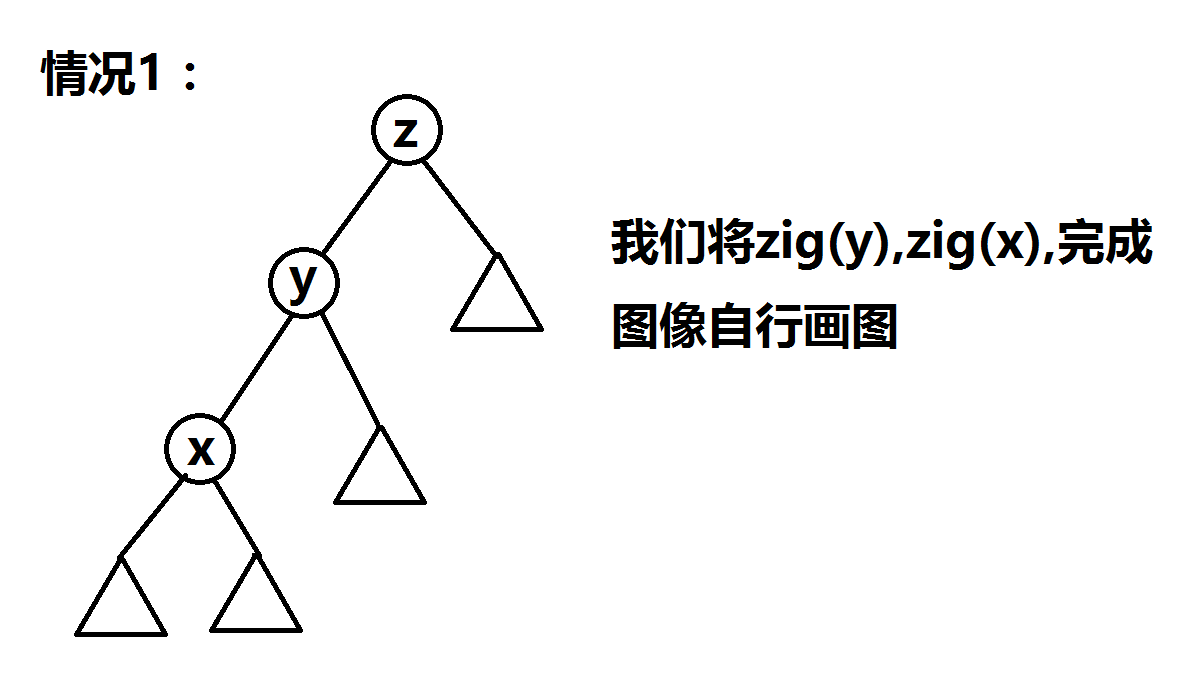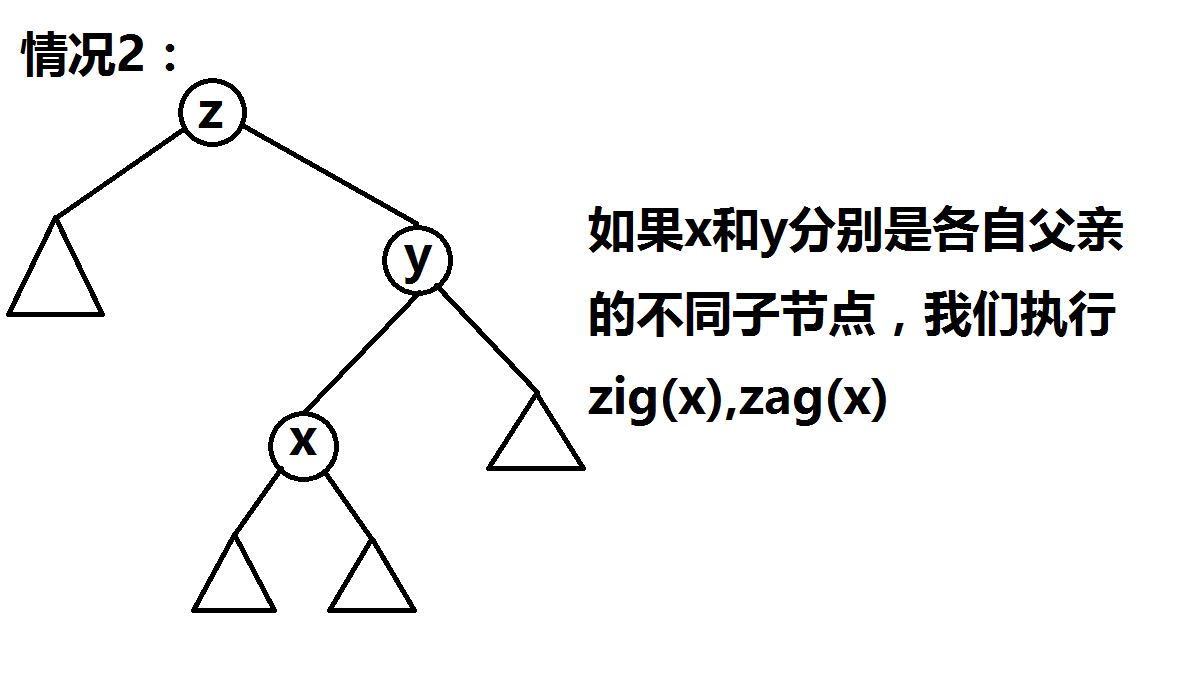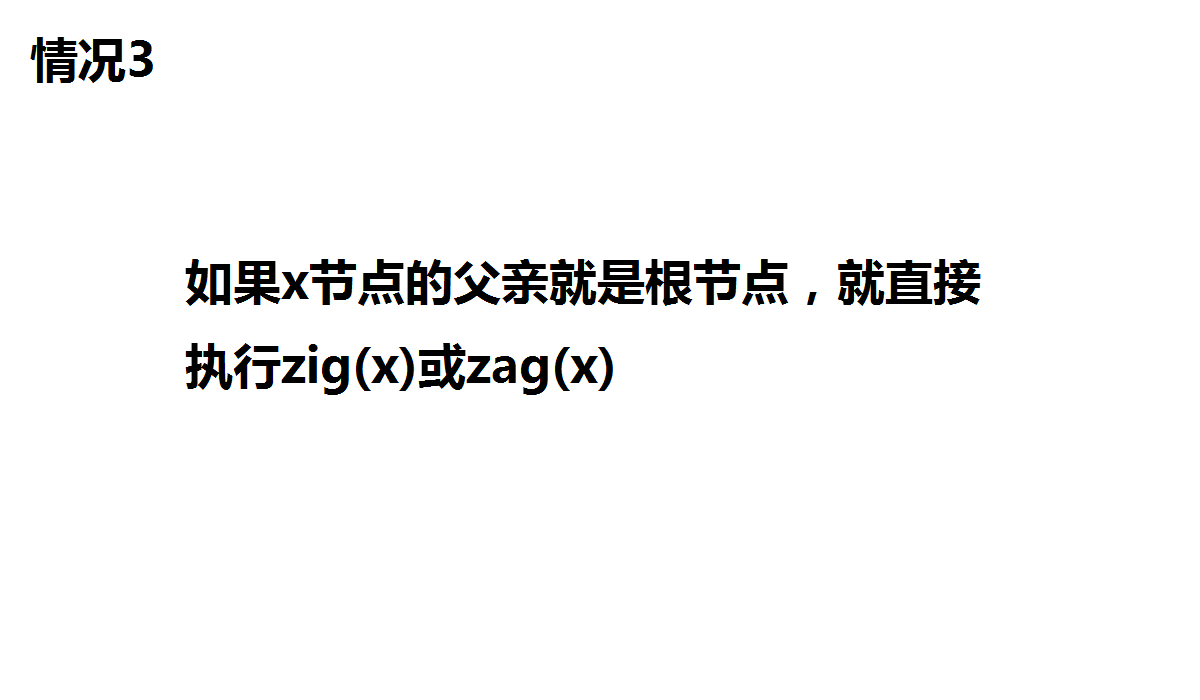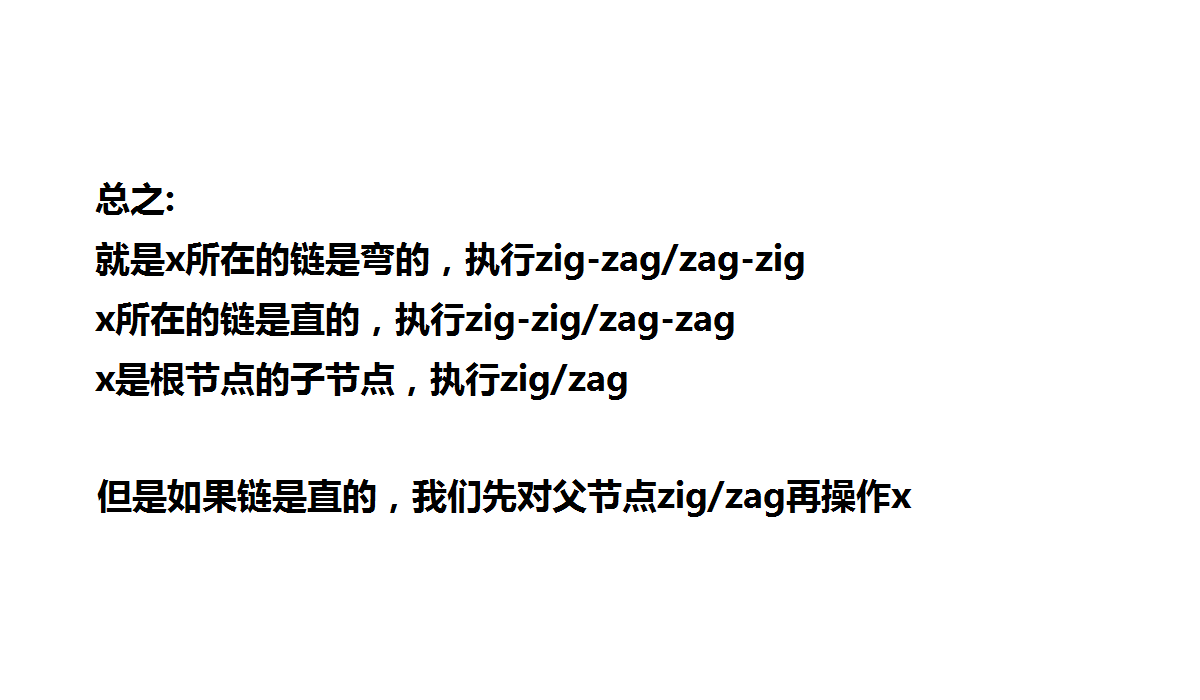#### 所以以下就是将 x 节点旋转到根节点的操作

/*
wson 为判断自己是父亲的哪个儿子的函数,，0 左 1 右
*/
inline bool wson(int node){return tree[tree[node].fa].s==node;}
inline void rot(int a)//有取反操作，可以很方便地执行 zig 或 zag 操作而不加判断
{
int f=tree[a].fa,g=tree[f].fa,p=wson(a),q=wson(f);
tree[f].s[p]=tree[a].s[!p],  tree[a].s[!p]=f,  tree[f].fa=a;//顺序很重要
if(tree[f].s[p])tree[tree[f].s[p]].fa=f;
if(tree[a].fa=g)tree[g].s[q]=a;
}
inline void splay(int node)
{
while(tree[node].fa)
if(!tree[tree[node].fa].fa)rot(node);//node 是根节点的子节点
else if(wson(node)!=wson(tree[node].fa))rot(node),rot(node);//链是弯的
else rot(tree[node].fa),rot(node);//链是直的
root=node;
}


## 3.Splay 的插入操作

/*

*/
//为了在新建节点的同时它父节点的儿子可以被更新，我们传入的是&node
void insrt(int x,int fa,int &node)
{
if(!node){node=++cnt,tree[node].x=x,tree[node].fa=fa,splay(node);return;}
if(x<tree[node].x)insrt(x,node,tree[node].s);
else if(x>tree[node].x)insrt(x,node,tree[node].s);
}


## 4.Splay 的前驱后继

 /*

*/
int nxt(int x,int node)
{
if(!node)return +1e8;
if(tree[node].x==x){splay(node);return x;}
if(tree[node].x>x)return min(nxt(x,tree[node].s),tree[node].x);
else return nxt(x,tree[node].s);
}
int pre(int x,int node)
{
if(!node)return -1e8;
if(tree[node].x==x){splay(node);return x;}
if(tree[node].x<x)return max(pre(x,tree[node].s),tree[node].x);
else return pre(x,tree[node].s);
}


## 5.Splay 节点的删除

/*

*/
void smax()
{
int a=root;
while(t[a].s)a=t[a].s;
splay(a);
}
int find(int x,int a)
{
while(a)
{
if(t[a].x==x)return a;
else if(t[a].x<x)a=t[a].s;
else a=t[a].s;
}
return a;
}
void del(int a)
{
splay(find(a,root));
int rs=t[root].s,ls=t[root].s;
if(rs==0){t[root].s=t[root].s=t[root].f=0,root=ls,t[root].f=0;return;}
if(ls==0){t[root].s=t[root].s=t[root].f=0,root=rs,t[root].f=0;return;}
t[root].s=t[root].s=0;//先将根和子节点的联系断开
t[rs].f=t[ls].f=0;
root=ls;
smax();//将左子树的最大值转到根
t[root].s=rs;//合并左右子树称为新的树
t[rs].f=root;
}


# 进阶 Splay 操作

## 2. 序列在 Splay 中的保存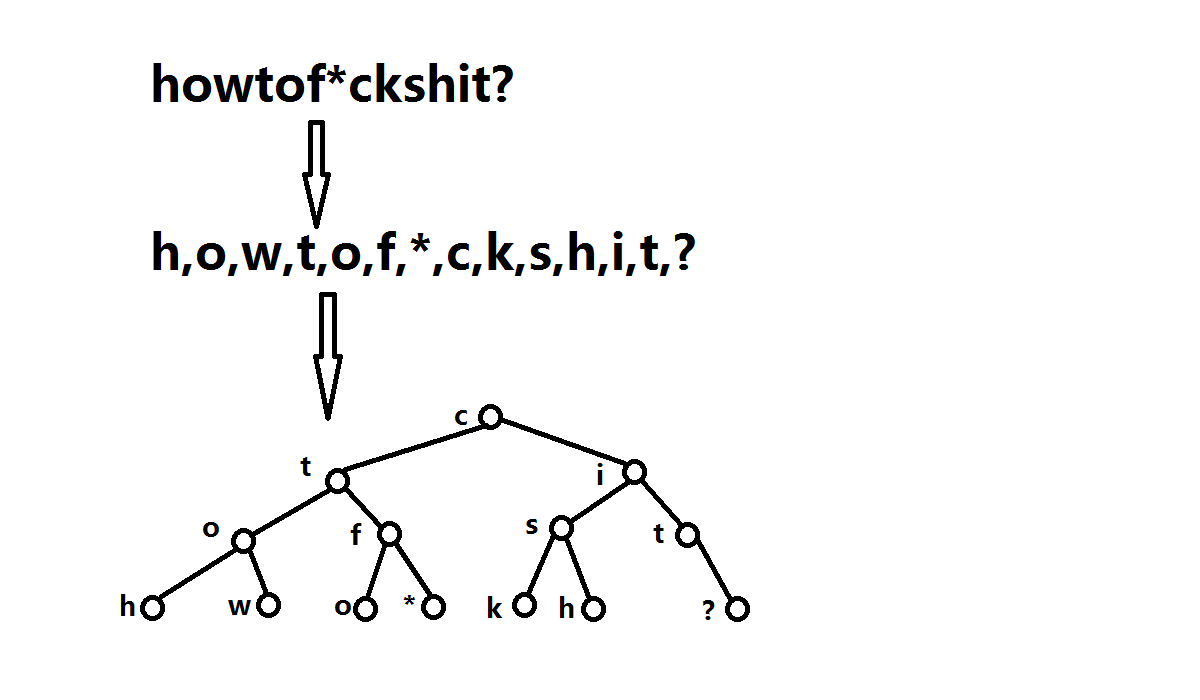## 4.Splay 中序列的反转

/*

*/
void pushdown(int node)
{
if(tree[node].lazy)
{
swap(tree[node].son,tree[node].son);
if(tree[node].son)tree[tree[node].son].lazy^=1;
if(tree[node].son)tree[tree[node].son].lazy^=1;
tree[node].lazy=0;
}
}